International
Tables for
Crystallography
Volume B
Reciprocal space
Edited by U. Shmueli

International Tables for Crystallography (2010). Vol. B, ch. 4.6, pp. 599-600   | 1 | 2 |

## Section 4.6.3.1.2. Diffraction symmetry

W. Steurera* and T. Haibacha

aLaboratory of Crystallography, Department of Materials, ETH Hönggerberg, HCI G 511, Wolfgang-Pauli-Strasse 10, CH-8093 Zurich, Switzerland
Correspondence e-mail:  walter.steurer@mat.ethz.ch

#### 4.6.3.1.2. Diffraction symmetry

| top | pdf |

The Laue symmetry group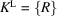of the Fourier module,is isomorphous to or a subgroup of one of the 11 3D crystallographic Laue groups leavinginvariant. The action of the point-group symmetry operators R on the reciprocal basis, can be written asThematricesform a finite group of integral matrices which are reducible, since R is already an orthogonal transformation in 3D physical space. Consequently, R can be expressed as pair of orthogonal transformationsin 3D physical and dD perpendicular space, respectively. Owing to their mutual orthogonality, no symmetry relationship exists between the set of main reflections and the set of satellite reflections.is the transpose ofwhich acts on vector components in direct space.

For thedirect-space (superspace) symmetry operator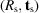and its matrix representation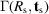on Σ, the following decomposition can be performed: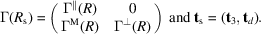is amatrix,is amatrix and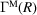is amatrix. The translation operator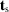consists of a 3D vectorand a dD vector. According to Janner & Janssen (1979),can be derived from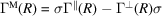.has integer elements only as it contains components of primitive-lattice vectors of, whereas σ in general consists of a rational and an irrational part: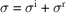. Thus, only the rational part gives rise to nonzero entries in. With the order of the Laue group denoted by N, one obtains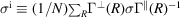, where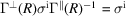, implying that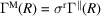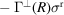and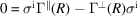.

#### Example

In the case of a 3D IMS with 1D modulationthematrixhas the components of the wavevector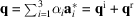.because for, q can only be transformed into. Corresponding to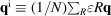, one obtains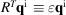(modulo). Therow matrixis equivalent to the difference vector betweenand(Janssen et al., 2004).

For a monoclinic modulated structure with point groupfor(unique axis) and satellite vector, withan irrational number, one obtains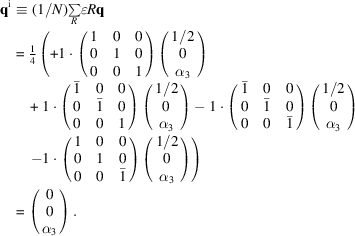From the relations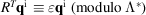, it can be shown that the symmetry operations 1 and 2 are associated with the perpendicular-space transformations, and m andwith. The matrixis given by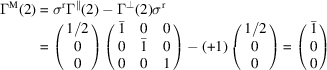for the operation 2, for instance.

The matrix representations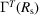of the symmetry operators R in reciprocalsuperspace decompose according to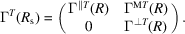Phase relationships between modulation functions of symmetry-equivalent atoms can give rise to systematic extinctions of different classes of satellite reflections. The extinction rules may include indices of both main and satellite reflections. A full list of systematic absences is given in the table ofsuperspace groups (Janssen et al., 2004). Thus, once point symmetry and systematic absences are found, the superspace group can be obtained from the tables in a way analogous to that used for regular 3D crystals. A different approach for the symmetry description of IMSs from the 3D Fourier-space perspective has been given by Dräger & Mermin (1996).

### References

Dräger, J. & Mermin, N. D. (1996). Superspace groups without the embedding: the link between superspace and Fourier-space crystallography. Phys. Rev. Lett. 76, 1489–1492.
Janner, A. & Janssen, T. (1979). Superspace groups. Physica A, 99, 47–76.
Janssen, T., Janner, A., Looijenga-Vos, A. & de Wolff, P. M. (2004). Incommensurate and commensurate modulated crystal structures. In International Tables for Crystallography, Vol. C, edited by E. Prince, ch. 9.8. Dordrecht: Kluwer Academic Publishers.